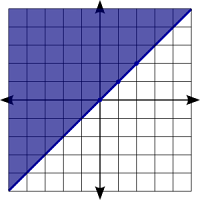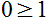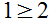## Sunday, December 30, 2007

### Math Facts Question Generator

Here it is I finally finished the the Math Facts Question Worksheet generator. With a few adjustments I was able to adapt most of the features of the Moodle Math Question generator to be able to create a printable version with an answer key. This program will create a set of math fact questions based on the users input parameters. At this point this version is limited to addition, subtraction, multiplication, and division.

Highlights:

• Vertical or horizontal problem formatting.
• Each time that the page is requested the order of the questions is changed.
• The answer key prints on a separate page.
• Customizable directions
• Custom key/worksheet identifier.
• Integer only answers for division problems.
• Positive answers for subtraction problems.
Check it out

## Tuesday, December 18, 2007

### Graphing website

While tutoring an Algebra 2 student I stumbled upon this website that looks pretty useful and seems to work pretty well. Thanks Zona.

EZ Graph @ http://id.mind.net/~zona/ezGraph/ezGraph.html

## Friday, December 14, 2007

### Graphing Systems of Equations

The goal of solving systems of equations is to determine if two or more lines intersect and if they do where do they intersect. This lesson will only deal with two lines.

First we need to determine how the lines of the two equations relate to each other. Do they intersect, are they parallel, or are they the same line. The mathematical ways of describing this is are they consistent, inconsistent, dependent, and independent. There are three options as shown in the picture below: To find the answer to the system you need to ask one or two questions depending on the problem.

Parallel lines: Independent and inconsistent

Question 1: First write the equation in slope intercept form. Then ask the question is the slope in each equation the same or different? If it is the same then they are parallel and you need to proceed to the next question. If it is different then the point at which the lines cross is the solution to the system and we are done.

Question 2: Since the slope is the same the lines are parallel. Now you need to ask the question "Are they the same line?" If the y-intercepts are the same then they are the same line and there is an infinite number of solutions. The line is the solution to the system of equations because it represents all of the points that work in both equations which are really the same equation just written differently.

If they are not the same then there is no solution, that is the lines do not cross.

Practice Problems:

Find the solution of the equations y = 2x + 4 and y = 0.5x - 2

First we recognize that the equations are in slope intercept form (y = mx + b) and that the slopes of each of the lines are different so we know that they will cross at some point. The next step is to graph the line. It is very important that your lines be accurate so I would recommend placing as many calculated points as possible on the graph for each line.

The solution is ( -4, -4 ). Make sure to check your answer by plugging the point into both problems.
y = 2x + 4
-4 = 2 ( -4) + 4
-4 = -8 + 4
-4 = -4

and

y = 0.5x - 2
-4 = 0.5 (-4) - 2
-4 = -2 - 2
-4 = 4

Lets try another example:
Solve the system of equations:
y= 4
2x - 3y = -6

First off notice that the second equation is in standard form and not slope intercept form. We need to rearrange it so that we can compare the slopes.
2x - 3y = -6
Subract 2x from both sides.
-3y = -2x - 6
Now divide both sides by -3
y = (2/3)x + 2

Again notice that the slopes are not the same so we know that they will cross at some point. Graph your line, making sure to plot lots of points to keep it accurate.The solution is ( 3, 4). Check your answer
y = 4
4 = 4. Remember that this equation doesn't care about the x value so it can be anything.

2x - 3y = -6. Always use the original problem in case you made a mistake when you were changing the problem to slope-intercept form.
2(3) - 3(4) = -6
6 - 12 = -6
-6 = -6

## Monday, December 10, 2007

### How to Graphing Inequalities in the Coordinate Plane.

Objective:
• Graph inequalities in a xy coordinate graph.

Assumptions:
• Ability to graph a line using the slope-intercept form (y = mx + b)

Concepts:

• The shaded area of a graph represents all of the coordinates that will work in a given equation.
• A solid edge of the shaded area means that the edge is part of the solutions to the equation.
• A dashed edge of the shaded area means that the edge of the graph is not part of the solutions.

Directions:
Graph the equationStep 1: Draw the graph just as you would y = x . This equations in slope intercept form would look like this. The 0 means that you will go through the origin, place a point there. Now use the slope to draw the rest of the line. From the origin go up one and to the right one and place another point. Repeat until you have several points.Now draw a solid line because the equation to be graphed is greater than or equal to. Your graph should now look like this:Step 2: Next shade everywhere above the line because the equation states that the y values are greater than or equal to the line for any given x value.Does the point ( 1, 2) work in the equation? yesDoes the point ( -1, 0) work in the equation? yesDoes the point ( 1, 0) work in the equation? noDoes the point ( 2, 1) work in the equation? noLets try another one.

Graph graph y > 2x + 3
Remember the steps: plot some points, draw the line (solid if equal to, dashed if greater than or less than), shade above with greater than, shade below with less than.
The line will cross the y axis as 3 then go up 2 and over 1 for the slope. Start by placing a point at 3 on the y axis. Next use the slope to place 2 more dots, then make a dashed line through the dots.
The equation uses the greater than inequality so it should be shaded above the line.

Now that we have the common ones out of the way lets look at the ones that may trip you up such as the ones with only one variable like y > 2 and x < -3.

Graph y > 2
Remember that is just a horizontal line. This is just a horizontal line that is shaded above the line and dashed because it is not equal to the line it is only greater than the line.

Graph x < -3

Remember that is just a vertical line. This is just a vertical line that is shaded to the left of the line and dashed because it is not equal to the line it is only less than the line. The x values on the left are less than the line.

Things to remember when graphing inequalities:
Solid line and shaded above the line.
Solid line and shaded below the line
> Dashed line and shaded above the line

y > # Horizontal line and shaded above the line
y < # Horizontal line and shaded below the line
x > # Vertical line and shaded on the right side of the line
x < # Vertical line and shaded on the left side of the line.

## Tuesday, December 4, 2007

### Random Student Selector

I my Algebra I class the other day I was using the Quizdom pick option to select my students to answer questions, during the class period 2 of my 11 students were selected 3 times while 2 others were not selected at all. Out of about 10 questions this wasn't very even percentages. One of the students Nathan J., who is also one of my computer programming students, and I were discussion this. We came up with a way to decrease the likelihood that someone would be selected once they have been selected. We chose this because if you eliminate the student until all have been selected the student will then mentally check out. With our solution the student can still be selected but the likelihood of them being selected twice in a row is reduced. Given enough time the Quizdom solution would be fine but if you are only asking 10 questions it is important that the student selection should be random and balanced. You can check out our free implementation of the student selector at http://www.aschool.us/moodle-scripts/

With this online implementation you simply enter a list of names, one per line. Go to the next page and start selecting students. Simply refreshing the page will select a new students name. The names are written in a large font so that it can be used in class.

An option that I have thought of adding would be to create a random list of the names so that it could printed to be used away from the computer and would still have the randomness for the students. If you are interested in this option please leave a comment. Since I have access to the Internet in my classroom I don't see this as a necessary feature for myself but if someone else would use it I could easily add it.